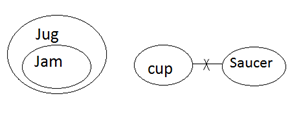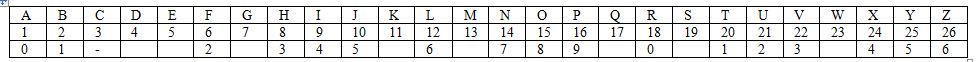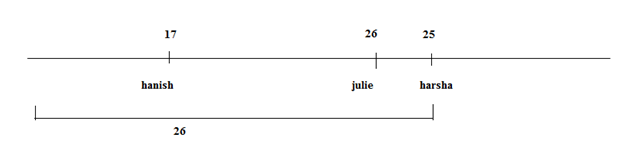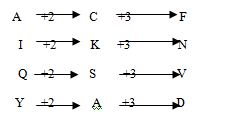# SSC CGL 2018 Practice Test Papers | Reasoning Ability (Day-1)

Dear Aspirants, Here we have given the Important SSC CGL Exam 2018 Practice Test Papers. Candidates those who are preparing for SSC CGL 2018 can practice these questions to get more confidence to Crack SSC CGL 2018 Examination.

[WpProQuiz 2607]

Click “Start Quiz” to attend these Questions and view Explanation

1). Find the odd one out

43, 49, 62, 80, 112, 154

1. 62
2. 80
3. 112
4. 154

2). 352:10:: 452: ?

100

20

11

15

3). Find the odd one out

1. Snake
2. Dinosaurus
3. Rhinocerous
4. Lizard

4). Statement:

All jam are jug. No cup is saucer.

Conclusion: i. Some cup are not jug is a possibility.

ii.No jam is saucer.

1. Only I follows
2. Only II follows
3. Both I and II
4. None of the above

5). If all the letters in an alphabet is coded from 0 to 9 excluding letters at prime number positions. What is the code for the word TRIUMPH. For example SCARCITY is coded as sc00c415.

1. t043m39
2. 1042m93
3. 5043693
4. t043793

6). Hanish is 17 from left end and Harsha is 25th from right end. Julie is in the middle of the line. The number of persons to the left of Harsha is 26. If Julie and Hanish interchange their positions what is the position of Hanish with respect to Harsha.

1. Immediate right
2. Immediate left
3. Second to the right
4. Second to the left

7). Which of the following assumptions can be made?

Statement: In 2016 flood, many people left their homes.

Assumptions: i. Houses are not strong.

ii). Government took measures to move to safe place.

1. Only I
2. Only II
3. Both I and II
4. Neither I nor II

8). In the following question select the missing number from the given series.

 23 5 7 11 18 16 15 27 ?
1. 25
2. 35
3. 13
4. 23

1. OSW
2. QSV
3. PRU
4. RTW

10). 4?3?5? 2. What is the order of arithmetic operators to be placed to get the value as 15.

1. +, -, x
2. x, +, –
3. -, x, +
4. -, +, x

43+2×3=49

49+3×4=62

62+4×5=82

82+5×6=112

112+6×7=154

3+5+2=10

4+5+2=11

Except c all are reptiles23+5+7=35

11+18+16=45

15+27+13=55